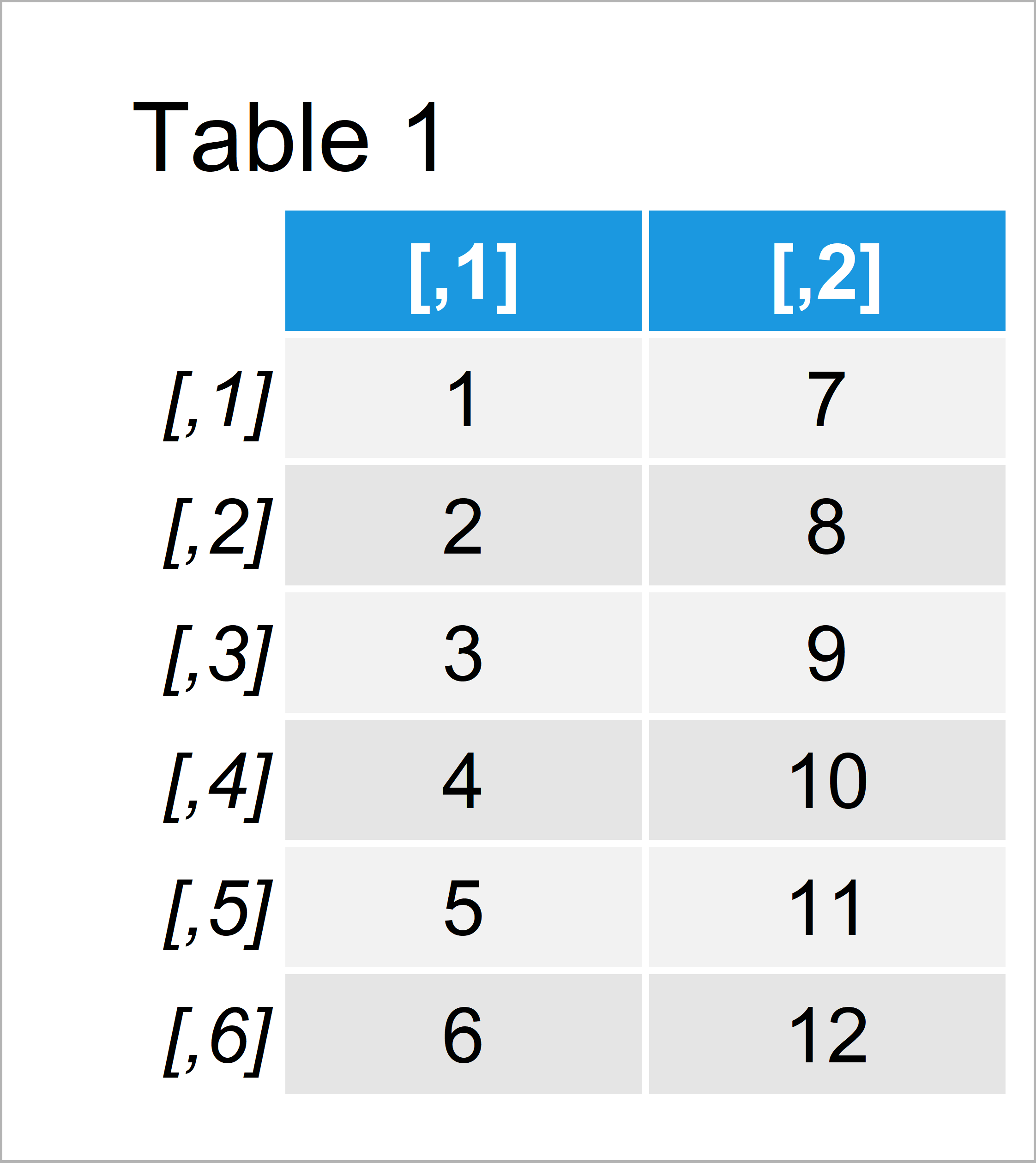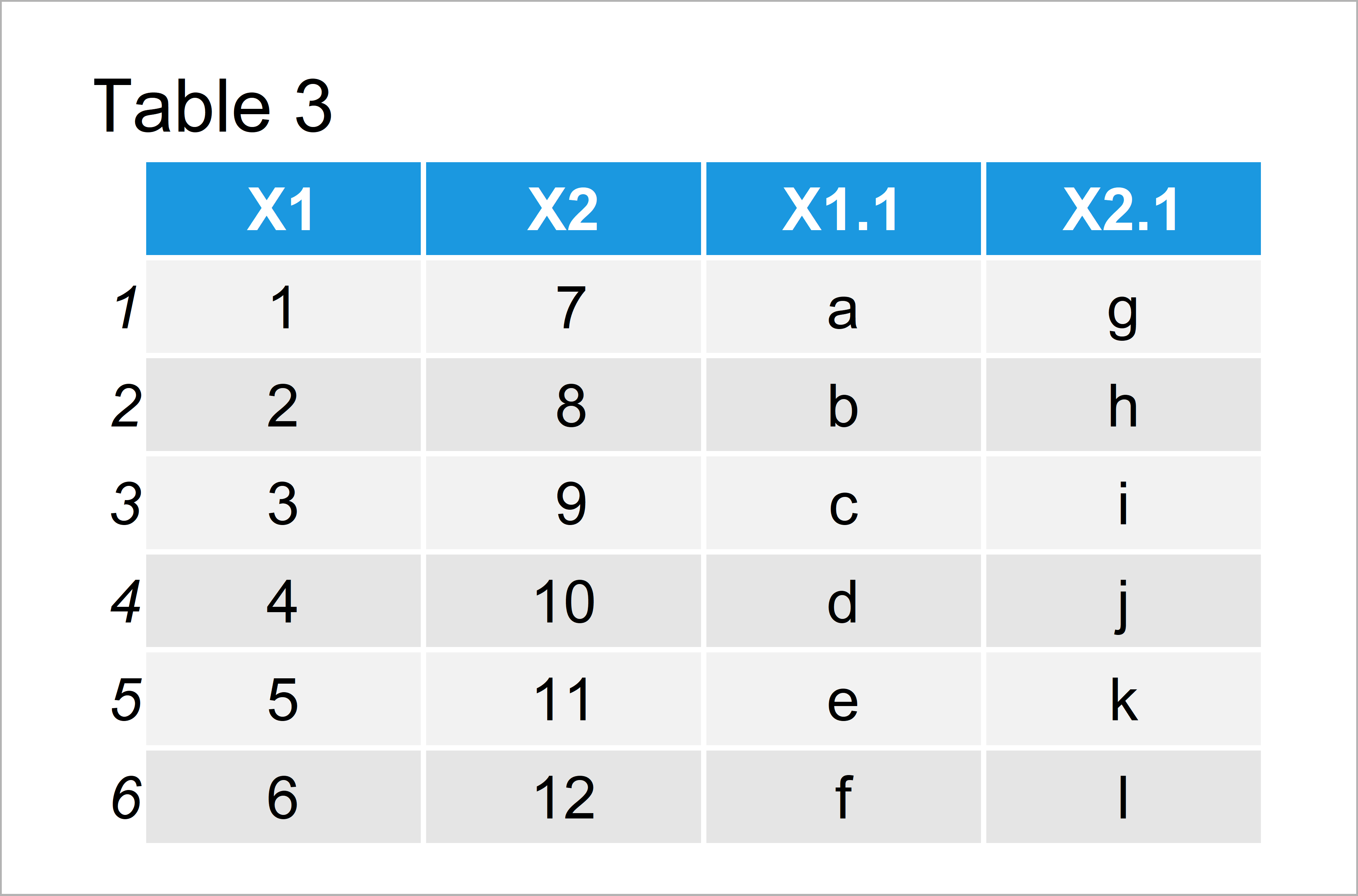# Merge Two Matrices by Columns in R (2 Examples)

In this tutorial you’ll learn how to merge the columns of two matrices in R.

The tutorial will contain this:

Sound good? Let’s get started:

## Exemplifying Data

We’ll use the following data as basement for this R programming language tutorial:

```mat1 <- matrix(1:12, ncol = 2)             # Create first example matrix
mat1                                       # Print first matrix```Table 1 visualizes the output of the RStudio console and shows that our first example matrix has six rows and two columns.

Let’s create another matrix:

```mat2 <- matrix(letters[1:12], ncol = 2)    # Create second example matrix
mat2                                       # Print second matrix```Table 2 shows the output of the previous R programming syntax – A second example matrix with six rows and two variables.

## Example 1: Merge Two Matrices Using data.frame() Function

In Example 1, I’ll show how to join our two matrices using the data.frame function in R.

Have a look at the following R syntax:

```merge1 <- data.frame(mat1, mat2)           # Merge matrices using data.frame()
merge1                                     # Print merged data frame```As shown in Table 3, we have created a data table containing the columns of our matrices mat1 and mat2.

Note that this data object has the data.frame class. Next; I’ll show how to convert this data frame back to the matrix class.

## Example 2: Convert Merged Data Frame to Matrix Class Using as.matrix() Function

This example explains how to apply the as.matrix function to convert our data frame to the matrix data type:

```merge2 <- as.matrix(merge1)                # Convert merged data frame to matrix
merge2                                     # Print merged matrix```As shown in Table 4, the previous R syntax has created a matrix consisting of our two input matrices mat1 and mat2.

## Video & Further Resources

Would you like to learn more about joining matrices? Then you might watch the following video of my YouTube channel. In the video, I explain the content of this tutorial.

Please accept YouTube cookies to play this video. By accepting you will be accessing content from YouTube, a service provided by an external third party.If you accept this notice, your choice will be saved and the page will refresh.

In addition, you might want to read the other posts of this website.

To summarize: In this tutorial you have learned how to join two matrix objects in the R programming language. Don’t hesitate to let me know in the comments section, if you have further questions.

Subscribe to the Statistics Globe Newsletter